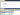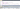Click on the banner to learn about and purchase my database training on Azure

# MySQL - How to convert time stored as an integer to a time column

Views: 5.972

Hello people,
Good night!

In this post today I will demonstrate how to convert stored integer time to a time column. I had the idea of ​​doing this post when a colleague DBA told me that he is running a system that uses the MySQL database and has a table where the programmer resolves to store time in an entire field, so that 09: 30 is stored with 930 and 21: 20 is stored as 2120, for example.

Unfortunately, this was not new to me and it motivated me to write this post, as it can help other people, whether DBA to work with this data or Developer, so that he uses datetime field or time to store this type. of information.

## Simulating the test base

So that you can simulate this problem at home, I decided to make this simple script available:

## Converting stored time as an INT column to TIME

After making this introduction and posting the test script, I will now demonstrate how to perform this integer-to-team conversion.

Using CONVERT
One quick way to do this is by using MySQL's CONVERT function to convert the number to TIME. If we try to do the conversion directly, MySQL will transform the data, but it will understand that the data is represented in the form mm: ss as shown below:Converting to seconds and then using the SEC_TO_TIME () function
Another way to do this is:

• Manually separate the time field value (LEFT (hora_int, CHAR_LENGTH (hora_int) - 2)
• Once the time value is obtained, the time obtained by 3600 (60 x 60) is multiplied to calculate the number of seconds that make up the time.
• Manually separate minute field value (RIGHT (hour_int, 2))
• Once the minute value is obtained, the minute obtained by 60 is multiplied to calculate the number of seconds that make up the minutes.
• Add the number of seconds of the hour to the number of seconds of minutes to calculate the total number of seconds.
• Once converted to seconds, we can use MySQL's SEC_TO_TIME () function to get the corresponding time from the calculated number of seconds.And now we can check out the summary solution:

I used the SELECT statement to display the final result, but you can quietly use UPDATE, converting from INT to TIME. In this case, I recommend creating a new column of type TIME, performing UPDATE, and if all is well, delete the column INT.

I hope you enjoyed the post!
Any questions, just leave in the comments.